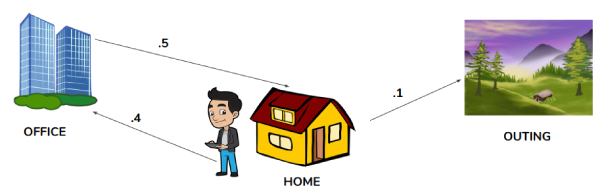• +91-9872993883
• +91-8283824812
• info@ris-ai.com

# Markov Chain¶

### Markov Chain¶

A stochastic process containing random variables, transitioning from one state to another depending on certain assumptions and definite probabilistic rules.## Markov Property ¶

A Markov chain is a stochastic process , but it differs from a general stochastic process in that a Markov chain must be "memory-less

Markov Property states that the calculated probability of a random process transitioning to the next possible state is only dependent on the current state and time and it is independent of the series of states that preceded it.### Transition Matrix ¶

Square matrix used to describe the transitions of a Markov chain. Each of its entries is a nonnegative real number representing a probability. It is also called a probability matrix, substitution matrix, Markov matrix or stochastic matrixIn :
import numpy as np
# open and read the data set
text = "Love python.Work python.Fast python.My python world.Machine leraning using python.Vast subject with many python library.It contain numpy panda and many other packages.One of the most used language in the world of artificial intelligence is python.When you work in python you will love it."
print(text)

Love python.Work python.Fast python.My python world.Machine leraning using python.Vast subject with many python library.It contain numpy panda and many other packages.One of the most used language in the world of artificial intelligence is python.When you work in python you will love it.

In :
split_text = text.split()
print(split_text)

['Love', 'python.Work', 'python.Fast', 'python.My', 'python', 'world.Machine', 'leraning', 'using', 'python.Vast', 'subject', 'with', 'many', 'python', 'library.It', 'contain', 'numpy', 'panda', 'and', 'many', 'other', 'packages.One', 'of', 'the', 'most', 'used', 'language', 'in', 'the', 'world', 'of', 'artificial', 'intelligence', 'is', 'python.When', 'you', 'work', 'in', 'python', 'you', 'will', 'love', 'it.']

In :
# work with generator
# here we are making pairs of keys and their followed words
def text_pairs(split_text):
for i in range(len(split_text)-1):
yield(split_text[i],split_text[i+1])
#print(split_text[i],split_text[i+1])

pair = text_pairs(split_text)
print(pair)
print(len(split_text))

<generator object text_pairs at 0x7f8f9a618360>
42

In :
text_dict = {}
for word_1,word_2 in pair:
if word_1 in text_dict.keys():
text_dict[word_1].append(word_2)
else:
text_dict[word_1]=[word_2]

In :
fword=np.random.choice(split_text)
while fword.islower():
fword=np.random.choice(split_text)

markov_chain=[fword]

In :
nword=20
for i in range(nword):
markov_chain.append(np.random.choice(text_dict[markov_chain[-1]]))

print(' '.join(markov_chain))

library.It contain numpy panda and many python library.It contain numpy panda and many python library.It contain numpy panda and many python

In [ ]:


In [ ]: